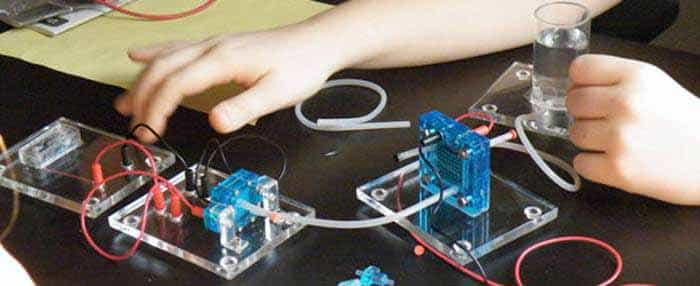Medical Sample papers

Calorimetry QuestionsCalorimeters and Calorimetry Physics Question Bank:

Ques: When vapour condenses into liquid
(a) It absorbs heat
(b) It liberates heat
(c) Its temperature increases
(d) Its temperature decreases
Ans. (b)

Ques: The saturation vapour pressure of water at 100°C is
(a) 739 mm of mercury
(b) 750 mm of mercury
(c) 760 mm of mercury
(d) 712 mm of mercury
Ans. (c)

Ques: Melting point of ice
(a) Increases with increasing pressure
(b) Decreases with increasing pressure
(c) Is independent of pressure
(d) Is proportional to pressure
Ans. (b)

Ques: The factor not needed to calculate heat lost or gained when there is no change of state is
(a) Weight
(b) Specific heat
(c) Relative density
(d) Temperature change
Ans. (c)

Ques: A quantity of heat required to change the unit mass of a solid substance, from solid state to liquid state, while the temperature remains constant, is known as
(a) Latent heat
(b) Sublimation
(c) Hoar frost
(d) Latent heat of fusion
Ans. (d)

Ques: If mass energy equivalence is taken into account, when water is cooled to form ice, the mass of water should
(a) Increase
(b) Remain unchanged
(c) Decrease
(d) First increase then decrease
Ans. (b)

Related: Genetic Engineering (Biology)

Ques: Amount of heat required to raise the temperature of a body through 1K is called its
(a) Water equivalent
(b) Thermal capacity
(c) Entropy
(d) Specific heat
Ans. (b)

Ques: If temperature scale is changed from °C to °F, the numerical value of specific heat will
(a) Increases
(b) Decreased
(c) Remains unchanged
(d) None of the above
Ans. (b)

Ques: When we rub our palms they gets heated but to a maximum temperature because
(a) Heat is absorbed by our palm
(b) Heat is lost in the environment
(c) Produced of heat is stopped
(d) None of the above
Ans. (b)

Ques: A body of mass 5 kg falls from a height of 30 metre. If its all mechanical energy is changed into heat, then heat produced will be
(a) 350 cal
(b) 150 cal
(c) 60 cal
(d) 6 cal
Ans. (a)

Related: GEEE Maths Question Papers

Ques: At atmospheric pressure, the water boils at 100°C. If pressure is reduced, it will boil at
(a) Higher temperature
(b) Lower temperature
(c) At the same temperature
(d) At critical temperature
Ans. (b)

Ques: The weight of a person is 60 kg. If he gets 105 calories heat through food and the efficiency of his body is 28%, then upto how much height he can climb (approximately)
(a) 100 m
(b) 200 m
(c) 400 m
(d) 1000 m
Ans. (b)

Ques: The temperature at which the vapour pressure of a liquid becomes equals to the external (atmospheric) pressure is its
(a) Melting point
(b) Sublimation point
(c) Critical temperature
(d) Boiling point
Ans. (d)

Ques: A liquid boils when its vapour pressure equals
(a) The atmospheric pressure
(b) Pressure of 76.0 cm column of mercury
(c) The critical pressure
(d) The dew point of the surroundings
Ans. (a)

Related: MCQs on Krebs Cycle for NEET

Ques: The mechanical equivalent of heat J is
(a) A constant
(b) A physical quantity
(c) A conversion factor
(d) None of the above
Ans. (c)

Ques: How many grams of a liquid of specific heat 0.2 at a temperature 40°C must be mixed with 100 gm of a liquid of specific heat of 0.5 at a temperature 20°C, so that the final temperature of the mixture becomes 32°C
(a) 175 gm
(b) 300 gm
(c) 295 gm
(d) 375 gm
Ans. (d)

Ques: A block of mass 100 gm slides on a rough horizontal surface. If the speed of the block decreases from 10 m/s to   5 m/s, the thermal energy developed in the process is
(a) 3.75 J
(b) 37.5 J
(c) 0.375 J
(d) 0.75 J
Ans. (a)

Ques: 5 g of ice at 0°C is dropped in a beaker containing 20 g of water at 40°C. The final temperature will be
(a) 32°C
(b) 16°C
(c) 8°C
(d) 24°C
Ans. (b)

Related: Problems on Straight Lines

Ques: The freezing point of the liquid decreases when pressure is increased, if the liquid
(a) Expands while freezing
(b) Contracts while freezing
(c) Does not change in volume while freezing
(d) None of these
Ans. (a)

Ques: 10 gm of ice at 0°C is mixed with 100 gm of water at 50°C. What is the resultant temperature of mixture
(a) 31.2°C
(b) 32.8°C
(c) 36.7°C
(d) 38.2°C
Ans. (d)

Ques: A hammer of mass 1kg having speed of 50 m/s, hit a iron nail of mass 200 gm. If specific heat of iron is 0.105 cal/gm°C and half the energy is converted into heat, the raise in temperature of nail is
(a) 7.1°C
(b) 9.2°C
(c) 10.5°C
(d) 12.1°C
Ans. (a)

Ques: Which of the following has maximum specific heat?
(a) Water
(b) Alcohol
(c) Glycerine
(d) Oil
Ans. (a)

Ques: Dry ice is
(a) Ice cube
(b) Sodium chloride
(c) Liquid nitrogen
(d) Solid carbon dioxide
Ans. (d)

Related: Quiz: Endocrine System

Ques: Calculate the amount of heat (in calories) required to convert 5 gm of ice at 0°C to steam at 100°C
(a) 3100
(b) 3200
(c) 3600
(d) 4200
Ans. (c)